1. SeatHow much m² of fabric do we need to sew a 50cm-shaped cube-shaped seat if 10% of the material we add to the folds?
2. The second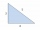The second angle of a triangle is the same size as the first angle. The third angle is 12 degrees larger than the first angle. How large are the angles?
3. Drilling machine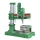A manufacturing firm purchased a heavy duty drilling machine. They were given two payment options: Option 1: Make a payment of \$46,000 immediately to settle the invoice for the machine. Option 2: Make a payment of \$21,500 immediately and the balance of
4. SummerjobThe temporary workers planted new trees. Of the total number of 500 seedlings, they managed to plant 426. How many percents did they meet the daily planting limit?
5. Prices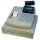The price of the product was increased by 35%. How many percents of the new price we have to make it cheaper so that its price is equal to the original price?
6. Drive to NJ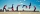Ed drove to New Jersey at 30mph. He drove back home in 3 hours at 50 mph. How many hours did it take Ed to drive to New Jersey?
7. Long bridge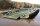Roman walked on the bridge. When he heard the whistle, he turned and saw running Kamil at the beginning of the bridge. If he went to him, they would meet in the middle of the bridge. Roman, however, rushed and so did not want to waste time returning 150m.
8. Dice and coinA number cube is rolled and a coin is tossed. The number cube and the coin are fair. What is the probability that the number rolled is greater than 2 and the coin toss is head?
9. Set of coordinatesConsider the following ordered pairs that represent a relation. {(–4, –7), (0, 6), (5, –3), (5, 2)} What can be concluded of the domain and range for this relation?
10. Equation with mixed fractions2 3/5 of 1430+? = 1900. How to do this problem
11. Pine's forest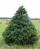There were so many pines in the forest that if they were sequentially numbered 1, 2, 3,. .. , would use three times more digits than the pine trees alone. How many pine trees were there in the forest?
12. Gasoline-oil ratioThe manufacturer of a scooter engine recommends a gasoline-oil fuel mixture ratio of 15 to 1. In a particular garage, we can buy pure gasoline and a gasoline-oil mixture, which is 75% gasoline. How much gasoline and how much of the gasoline-oil mix do w
13. Intersect and conjuctionLet U={1,2,3,4,5,6} A={1,3,5} B={2,4,6} C={3,6} Find the following. 1. )AUB 2. )A'UB'
14. One side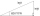One side is 36 long with a 15° incline. What is the height at the end of that side?
15. Two math problems1) The sum of twice a number and -6 is nine more than the opposite of that number. Find the number. 2) A collection of 27 coins, all nickels, and dimes, is worth \$2.10. How many of each coin are there? The dime, in United States usage, is a ten-cent coi
16. Car repair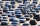John bought a car for a certain sum of money. He spent 10% of the cost to repairs and sold the car for a profit of Rs. 11000. How much did he spend on repairs if he made a profit of 20%?
17. Working aloneTom and Chandri are doing household chores. Chandri can do the work twice as fast as Tom. If they work together, they can finish the work in 5 hours. How long does it take Tom working alone to do the same work?55%+36%+88%+71%+100=63% what is whole (X)? Percents can be added directly together if they are taken from the same whole, which means they have the same base amount. .. . You would add the two percentages to find the total amount.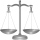Adelka, Barunka, and Cecilka are weight in pairs. Adelka with Barunka weighs 98 kg, Barunka with Cecilka 90 kg and Adelka with Cecilka 92 kg. How much does each of them weigh?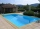It is possible to fill the pool with three tributaries. The first would take 12 hours, the second 15 hours, and the third 20 hours. The day before the summer season began, the manager opened all three tributaries simultaneously. How long did it take to fi